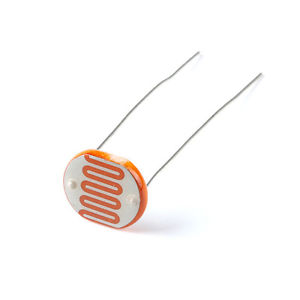# How to use a photoresistor with Arduino## INTRODUCTION

A photoresistor known as LDR (Light Decreasing Resistance, or light-dependent resistor) is an active component that decreases resistance when receiving light on its sensitive surface.

## APPLICATIONS

The photoresistor is used to detect the intensity of light in driverless cars. Night lights automatically turn on when the intensity of light detected by this sensor is below a threshold value.

## WIRING DIAGRAM

VCC – 5V

GND – GND

Analog Pin A0 and Digital Pin 12 are used## CODE

```int photopin = A0;
int ledpin = 12;

int value;

void setup() {
// put your setup code here, to run once:
Serial.begin(9600);
pinMode(photopin,INPUT);
pinMode(ledpin, OUTPUT);
}

void loop() {
// put your main code here, to run repeatedly:

if (value > 25){
digitalWrite(ledpin, LOW);

}
else {
digitalWrite(ledpin, HIGH);
}
}
int photopin = A0;
int ledpin = 12;

int value;

void setup() {
// put your setup code here, to run once:
Serial.begin(9600);
pinMode(photopin,INPUT);
pinMode(ledpin, OUTPUT);
}

void loop() {
// put your main code here, to run repeatedly:

if (value > 25){
digitalWrite(ledpin, LOW);

}
else {
digitalWrite(ledpin, HIGH);
}
}
```

## END RESULT

Put your finger over the photoresistor and the LED will light up.

```